# Irrigation Frequency

This calculates the maximum interval allowed between irrigations. This is dependent on soil type, root zone depth, and the water use rate of the crop.

## Irrigation Frequency

Soil's Available Water Holding Capacity:
Root Zone Depth:
Management Allowable Depletion:
Crop Water Use Rate:
Practical Use Irrigation Frequency:

### The Equation

This is the formula this calculator uses to determine the suggested irrigation frequency.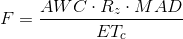Where: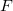= Suggested irrigation frequency (day)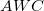= Soil's available water holding capacity (in/ft)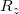= Root Zone Depth (ft)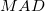= Management Allowable Depletion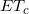= Crop water use rate (in/day)

Reference: Washington State University